# OECD Business confidence Index(BCI) data analysis 3 - time-series chart using ggplot() + geom_line()Photo by NASA on Unsplash

This post is following of above post.

Let's see time-series for bci average.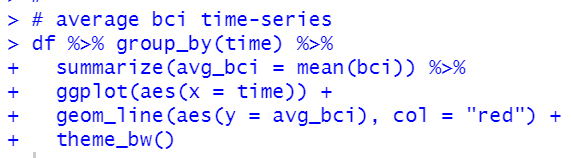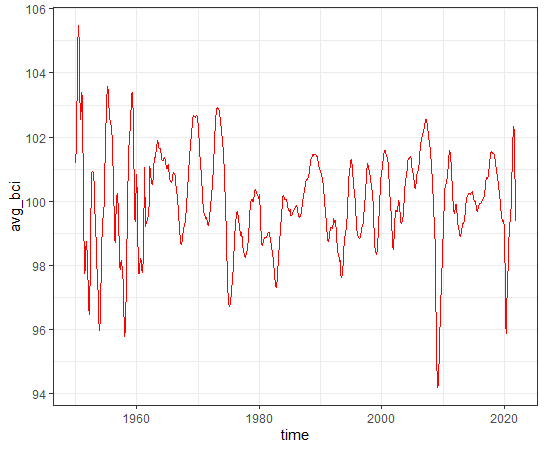Let's see time-series for good average.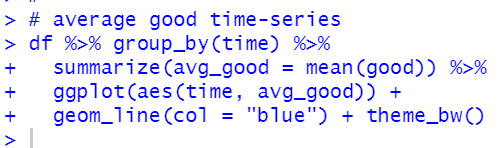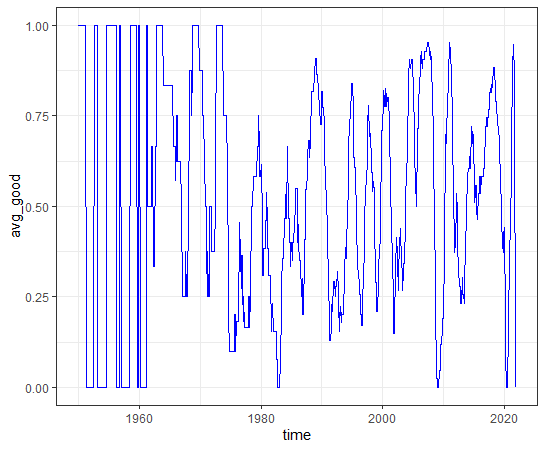average bci and average good are both very volatile.

what is the correlation for both?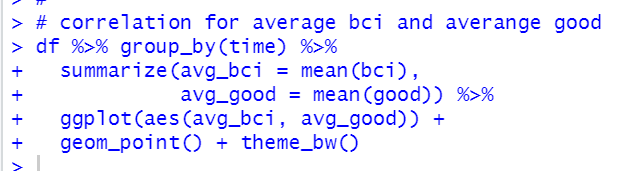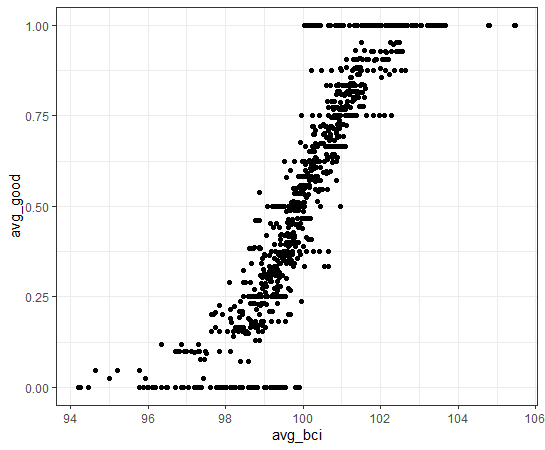Of course, we see positive correlation.

Let's see by region time series.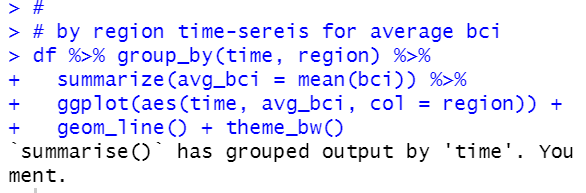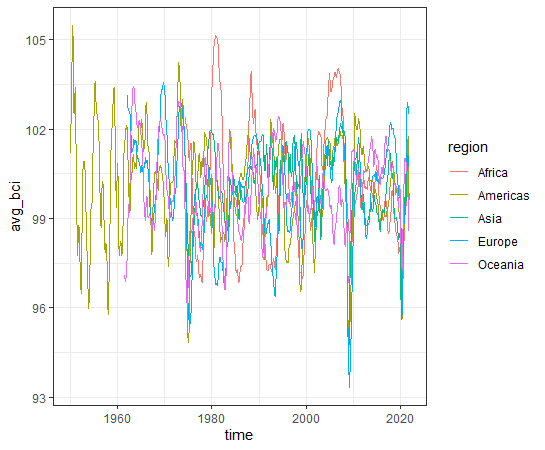It is too complicated to understand.

Let's see average good time-series by region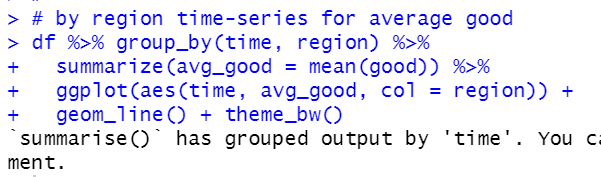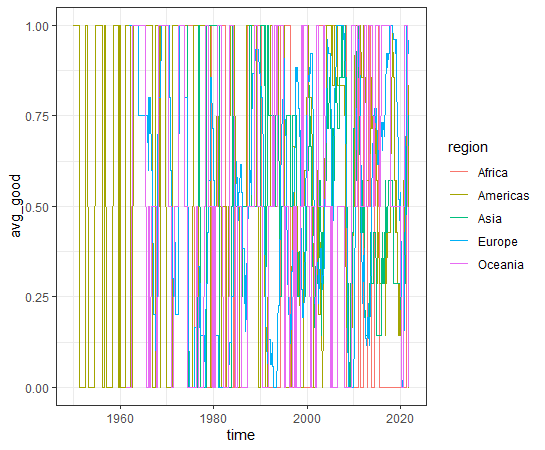This chart is also too complicated to understand, interpret.

All right, let's focus overall average now. So I will make overall average dataframe.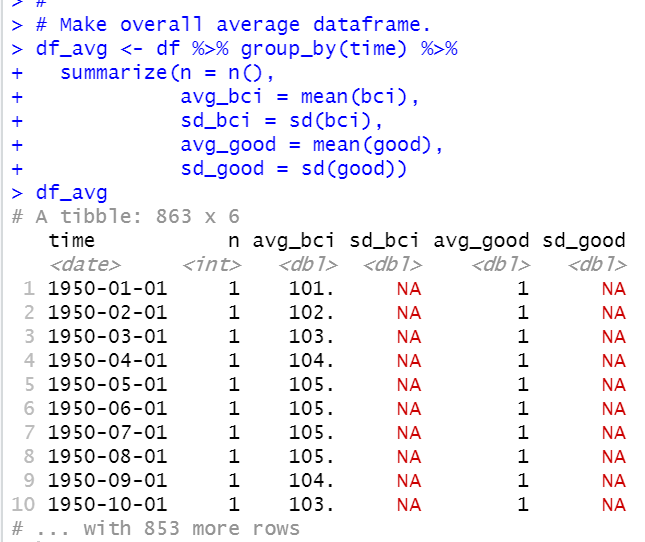If number of observations is only 1, standard deviations cannot be calculated.

We see 1950-01-01 and other time has NA.

I omit those observations.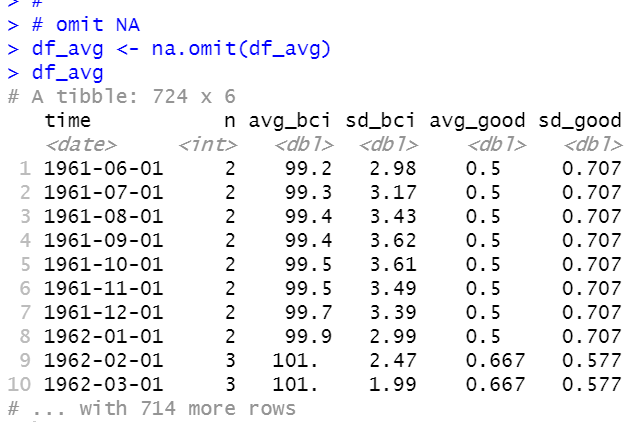Let's see summary of df_avg dataframe.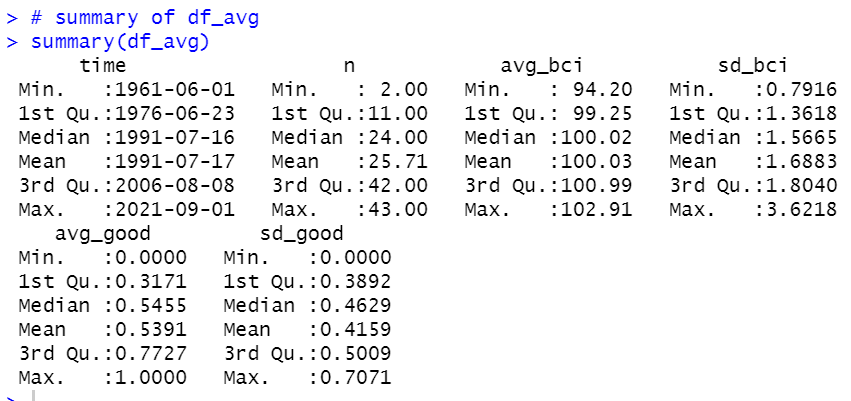All right, let's make those variables time-series charts.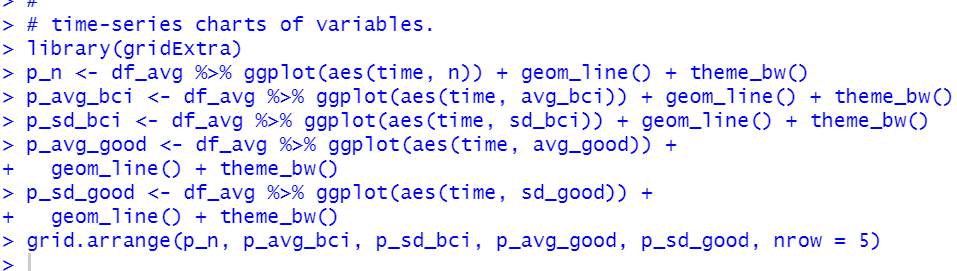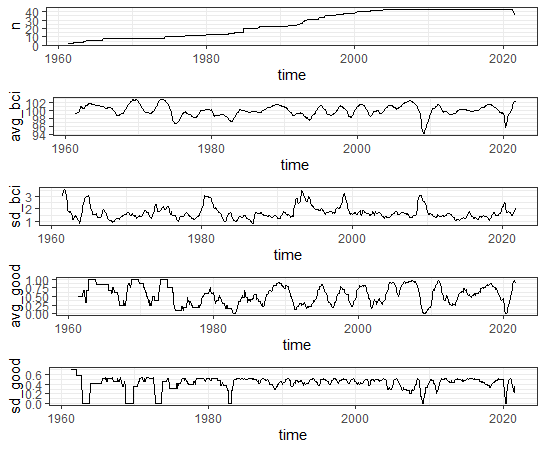It seems interesting.

That's it. Thank you!

Next post is